# Samacheer Kalvi 10th Maths Book Solutions Chapter 3 | Algebra Answers For Tamil Nadu Board

Samacheer Kalvi 10th Maths Book Solutions Chapter 3 – Algebra is available here. The Samacheer Kalvi 10th Maths book answers of Chapter 3, available at BYJU’S, contain step by step explanations designed by our Mathematics experts. All these important questions are based on the new pattern prescribed by the Tamil Nadu board. Students can also get the solutions of other chapters on Samacheer Kalvi 10th Maths solutions. Solving these Samacheer Kalvi Class 10 Maths Book Chapter 3 Solutions for practice is the best way to get acquinted with answering various question of different difficulty level.

Chapter 3 of the Samacheer Kalvi 10th Maths guide will help the students to solve problems related to simultaneous linear equations in two and three variables, GCD and LCM of polynomials, Relationship between LCM and GCD, rational expressions and its reduction, excluded value, operations of rational expressions, square root of polynomials, quadratic equations, solving quadratic equations, roots of a quadratic equation, the relation between roots and coefficients of a quadratic equation, quadratic graphs, matrices and their types, operations on matrices and their properties.

### Samacheer Kalvi 10th Maths Chapter 3: Algebra Book Exercise 3.1 Questions and Solutions

Question 1: Discuss the nature of the solution of the following system of equations:

(y + z) / 4 = (z + x) / 3 = (x + y) / 2 ; x + y + z = 27

Solution:

(y + z) / 4 = (z + x) / 3

3(y + z) = 4(z + x)

3y + 3z = 4z + 4x

4x – 3y + 4z – 3z = 0

4x – 3y + z = 0 — (1)

(z + x) / 3 = (x + y) / 2

2(z + x) = 3(x + y)

2z + 2x = 3x + 3y

3x – 2x + 3y – 2z = 0

x + 3y – 2z = 0 —- (2)

x + y + z = 27 —-(3)

(1) + (2)

4x – 3y + z = 0

x + 3y – 2z = 0

——————–

5x – z = 0 —- (4)

(1) – 3 * (3)

4x – 3y + z = 0

3x + 3y + 3z = 81

——————–

7x + 4z = 81 — (5)

4 * (4) + (5)

20x – 4z = 0

7x + 4z = 81

—————

27x = 81

x = 81 / 27 = 3

Therefore, the equations will have a unique solution.

By finding the solution further,

(4) => 7x + 4z = 81

7(3) + 4z = 81

21 + 4z = 81

4z = 81 – 21

4z = 60

z = 15

(1) => 4x – 3y + z = 0

4(3) – 3y + 15 = 0

12 + 15 – 3y = 0

-3y = 27

y = -9

### Samacheer Kalvi 10th Maths Chapter 3: Algebra Book Exercise 3.2 Questions and Solutions

Question 1: Find the

[i] GCD of x4 + 3x3 −x −3, x3 + x2 − 5x + 3

[ii] LCM of 4x2y, 8x3y2

Solution:

[i] To find the GCD of x4 + 3x3 −x −3, x3 + x2 − 5x + 3

Let f(x) = x4 + 3x3 −x −3

g(x) = x3 + x2 − 5x + 3

The degree of the polynomial f(x) is greater than g(x).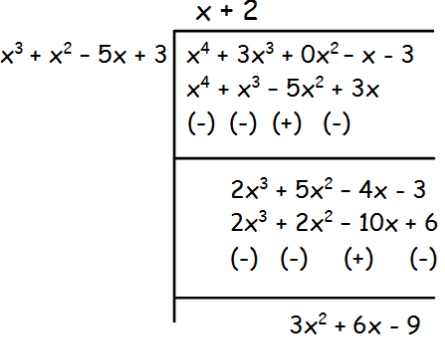The remainder is 3(x2 + 2x – 3), which is not equal to 0.

So, divide x3 + x2 − 5x + 3 by x2 + 2x – 3 by leaving the remainder.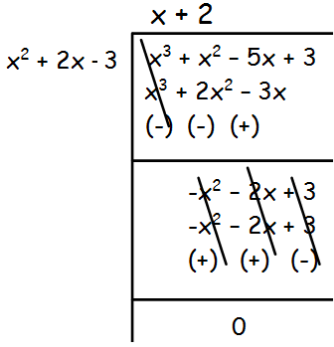Remainder 0 is obtained by dividing the given polynomial by x2 + 2x – 3.

Hence the GCD is x2 + 2x – 3.

[ii] To find the LCM of 4x2y, 8x3y2

4x2y = 2 ⋅ 2 ⋅ x2 ⋅ y = 22 ⋅ x2 ⋅ y

8x3y2 = 2 ⋅ 2 ⋅ 2⋅ x3 ⋅ y2 = 23 ⋅ x3 ⋅ y2

The highest power for 2 is 22, x is x3 and y is y2.

LCM = 23⋅ x3 ⋅ y2 = 8x3y2

### Samacheer Kalvi 10th Maths Chapter 3: Algebra Book Exercise 3.3 Questions and Solutions

Question 1: Find the GCD of the following polynomials: 12(x4 -x3) and 8(x4 − 3x3 + 2x2), given that LCM is 24x3 (x – 1) (x – 2).

Solution:

Let f(x) = 12(x4 -x3), g(x) = 8(x4 − 3x3 + 2x2)

LCM = 24x3 (x – 1) (x – 2)

f(x) = 12(x4 – x3)

f(x) = 12x3(x – 1)

g(x) = 8(x4 − 3x3 + 2x2)

g(x) = 8x2(x2 – 3x + 2)

GCD = 24x3(x -1)(x -2)

f (x) * g(x) = LCM * GCD

GCD = [f(x) × g(x)] / LCM

GCD = [12x3(x – 1) 8x2(x2 – 3x + 2)] / 24x3(x -1)(x -2)

GCD = 4x2(x-1)

### Samacheer Kalvi 10th Maths Chapter 3: Algebra Book Exercise 3.4 Questions and Solutions

Question 1: Reduce the following rational expression to its lowest form: (x2 – 1) / (x2 + x)

Solution:

(x2 – 1) / (x2 + x)

x2 – 1 = (x + 1) (x – 1)

(x2 + x) = x(x + 1)

= (x + 1) (x – 1) / x(x + 1)

= (x – 1) / x

### Samacheer Kalvi 10th Maths Chapter 3: Algebra Book Exercise 3.5 Questions and Solutions

Question 1: If x = (a2 + 3a – 4) / (3a2 – 3) and y = (a2 + 2a – 8) / (2a2 – 2a – 4) find the value of x2y-2.

Solution:

x = (a2 + 3a – 4) / (3a2 – 3)

= (a + 4)(a – 1) / 3(a2 – 1)

= (a + 4)(a – 1) / 3(a + 1)(a – 1)

= (a + 4) / 3(a + 1)

y = (a2 + 2a – 8) / (2a2 – 2a – 4)

= (a + 4)(a – 2) / 2(a – 2)(a + 1)

= (a + 4) / 2(a + 1)

x2y-2 = x2 / y2 = (x / y)2

= {[(a + 4) / 3(a + 1)] / [(a + 4) / 2(a + 1)]}2

= { [(a + 4) / 3(a + 1)] ⋅ [2(a + 1) / (a + 4)] }2

= (2 / 3)2

= 4 / 9

### Samacheer Kalvi 10th Maths Chapter 3: Algebra Book Exercise 3.6 Questions and Solutions

Question 1: Which rational expression should be subtracted from (x2 + 6x + 8) / (x3 + 8) to get 3 / (x2 – 2x + 4)?

Solution:

Let p(x) be the rational expression that should be subtracted.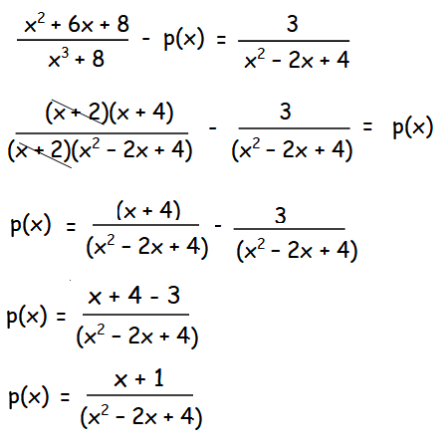### Samacheer Kalvi 10th Maths Chapter 3: Algebra Book Exercise 3.7 Questions and Solutions

Question 1: Find the square root of the rational expression: 400 x4 y12 z16 / 100 x8 y4 z4.

Solution:

= √400 x4 y12 z16 / 100 x8 y4 z4

= √(4 y8 z12 / x4)

= |(2 y4 z6) / x2|

### Samacheer Kalvi 10th Maths Chapter 3: Algebra Book Exercise 3.8 Questions and Solutions

Question 1: Find the values of a and b if the polynomial is a perfect square: 4x4 − 12x3 + 37x2 + bx + a

Solution: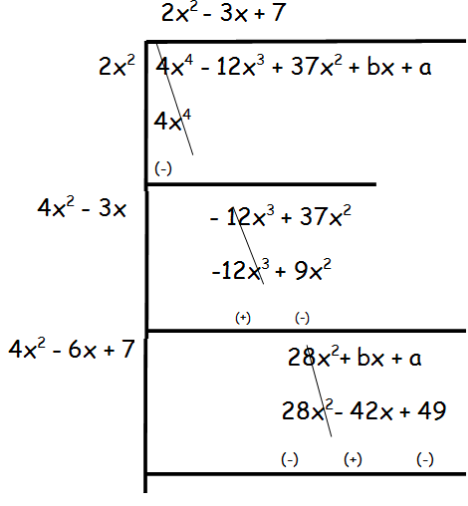By equating the coefficients of x, b = -42

By equating the constant terms, a = 49

Hence, a = 49 and b = -42.

### Samacheer Kalvi 10th Maths Chapter 3: Algebra Book Exercise 3.9 Questions and Solutions

Question 1: Find the sum and product of the roots for the quadratic equation: x2 + 3x −28 = 0

Solution:

By comparing the given equation x2 + 3x −28 = 0, with ax2 + bx + c = 0, a = 1, b = 3 and c = -28

Sum of zeroes (α + β) = -3 / 1 = -3

Product of zeroes (αβ) = -28 / 1 = -28

Hence, the sum and product of zeroes of the given quadratic equation are -3 and -28 respectively.

### Samacheer Kalvi 10th Maths Chapter 3: Algebra Book Exercise 3.10 Questions and Solutions

Question 1: The number of volleyball games that must be scheduled in a league with n teams is given by G (n) = (n2 – n) / 2 where each team plays with every other team exactly once. A league schedules 15 games. How many teams are in the league?

Solution:

Number of games scheduled = 15

(n2 – n) / 2 = 15

n2 – n = 30

n2 – n – 30 = 0

n2 – 6n + 5n – 30 = 0

n(n -6) + 5(n – 6) = 0

(n – 6) (n + 5) = 0

n – 6 = 0 and n + 5 = 0

n = 6 and n = -5

The number of teams cannot be a negative number.

Hence, 6 teams are in the league.

### Samacheer Kalvi 10th Maths Chapter 3: Algebra Book Exercise 3.11 Questions and Solutions

Question 1: Solve the quadratic equation by the formula method: 36y2 −12ay + (a2 −b2) = 0

Solution:

By comparing the given quadratic equation with the general form of quadratic equation,

ax2 + bx + c = 0,

a = 36, b = -12a and c = (a2 −b2)

x = [-b ± √b2 – 4ac] / 2a

x = [-(-12a) ± √(-12a)2 – 4(36)(a2 −b2)] / 2(36)

x = [12a ± √(144a2 – 144a2 + 144b2)] / 72

x = [12a ± √144b2] / 72

x = [12a ± 12b] / 72

x = 12(a ± b) / 72

x = (a ± b) / 6

x = (a + b) / 6

x = (a – b) / 6

Therefore, the solution is {(a + b) / 6, (a – b) / 6}.

### Samacheer Kalvi 10th Maths Chapter 3: Algebra Book Exercise 3.12 Questions and Solutions

Question 1: A girl is twice as old as her sister. Five years hence, the product of their ages (in years) will be 375. Find their present ages.

Solution:

Let x be the age of sister and 2x be the age of the girl

Five years hence,

Age of sister = x + 5

Age of girl = 2x + 5

Product of their ages = 375

(x + 5)(2x + 5 ) = 375

2x2 + 5x + 10x + 25 – 375 = 0

2x2 + 15x – 350 = 0

2x2 – 20x + 35x – 350 = 0

2x(x – 10) + 35(x -10) = 0

(2x + 35)(x – 10) = 0

x = 10

Hence, the age of the sister is 10 years, age of girl = 2(10) = 20 years.

### Samacheer Kalvi 10th Maths Chapter 3: Algebra Book Exercise 3.13 Questions and Solutions

Question 1: If the roots of (a − b)x2 + (b − c)x + (c − a) = 0 are real and equal, then prove that b, a, c are in arithmetic progression.

Solution:

By comparing the given quadratic equation with the general form of the quadratic equation, ax2 + bx + c = 0,

a = a – b, b = b – c and c = c – a

If the roots are real and equal, then Δ = 0

Δ = b2 – 4ac

(b – c)2 – 4(a – b)(c – a) = 0

b2 + c2 – 2bc – 4(ac – a2 – bc + ab) = 0

b2 + c2 – 2bc – 4ac + 4a2 + 4bc – 4ab = 0

b2 + c2 + 2bc – 4ac + 4a2 – 4ab = 0

4a2 + b2 + c2 – 4ab + 2bc – 4ac = 0

(2a)2 + (-b)2 + (-c)2 + 2(2a)(-b) + 2(-b)(-c) + 2(2a)(-c) = 0

(2a – b – c)2 = 0

2a = b + c

Hence a, b and c are in arithmetic progression.

Samacheer Kalvi 10th Maths Chapter 3: Algebra Book Exercise 3.14 Questions and Solutions

Question 1: If α, β are the roots of 7x2 + ax + 2 = 0 and if β − α = −13 / 7. Find the values of a.

Solution:

7x2 + ax + 2 = 0

a = 7, b = a and c = 2

Sum of roots (α + β) = -b / a = -a / 7

Product of roots (α β) = c / a = 2 / 7

β − α = −13 / 7

-(α – β) = -13 / 7

(α – β) = -13 / 7

√(α + β)2 – 4αβ = -13 / 7

(α + β)2 – 4αβ = (-13 / 7)2

(-a / 7)2 – 4(2 / 7) = 169 / 49

(a2 / 49) – (8 / 7) = 169 / 49

a2 – 56 = 169

a2 = 225

a = ±15

Samacheer Kalvi 10th Maths Chapter 3: Algebra Book Exercise 3.15 Questions and Solutions

Question 1: Graph the quadratic equation and state the nature of solutions: x2 − 9x + 20 = 0

Solution:

Draw the graph for the function y = x2 − 9x + 20

Taking random values for x, find the corresponding value of y.

 x x2 -9x 20 y -5 25 45 20 90 -4 16 36 20 72 -3 9 27 20 56 -2 4 18 20 42 -1 1 9 20 30 0 0 0 20 20 1 1 -9 20 12 2 4 -18 20 6 3 9 -27 20 2 4 16 -36 20 0 5 25 -45 20 0

To find the x-coordinate of the vertex of the parabola, use the formula x = -b / 2a,

x = -(-9) / 2(1) = 9 / 2

By putting the value of x = 9 / 2, the value of y is obtained.

y = (9 / 2)2 – 9(9 / 2) + 20

y = (81 / 4) – (81 / 2) + 20

y = (81 – 162 + 80) / 4 = -1 / 4

Vertex (9 / 2, – 1 / 4)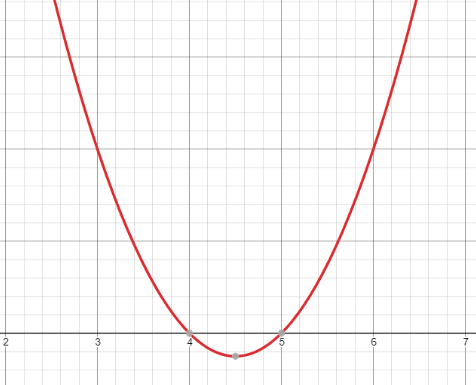The graph of the parabola intersects the x-axis at two distinct points.

Hence, it has real and unequal roots.

Samacheer Kalvi 10th Maths Chapter 3: Algebra Book Exercise 3.16 Questions and Solutions

Question 1: If a matrix has 18 elements, what are the possible orders it can have? What if it has 6 elements?

Solution:

Possible orders of matrices having 18 elements are 1 x 18, 18 x 1, 6 x 3, 3 x 6 , 2 x 9, 9 x 2.

Possible orders of matrices having 6 elements are 1 x 6, 6 x 1, 2 x 3, 3 x 2.

Samacheer Kalvi 10th Maths Chapter 3: Algebra Book Exercise 3.17 Questions and Solutions

Question 1: If

$$\begin{array}{l}A=\begin{bmatrix} 1 &9 \\ 3&4 \\ 8 & -3 \end{bmatrix}\end{array}$$
and
$$\begin{array}{l}B=\begin{bmatrix} 5 &7 \\ 3&3 \\ 1 & 0 \end{bmatrix}\end{array}$$
, then verify that A + B = B + A.

Solution:

[i] A + B =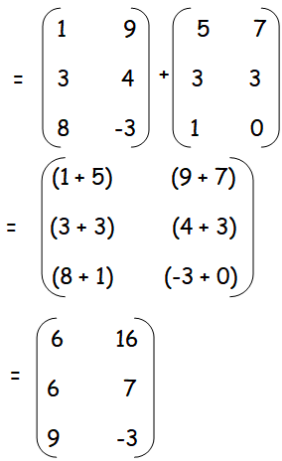B + A =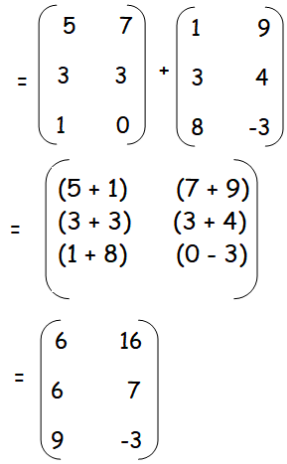So, A + B = B + A.

Samacheer Kalvi 10th Maths Chapter 3: Algebra Book Exercise 3.18 Questions and Solutions

Question 1: If A is of order p x q and B is of order q x r what is the order of AB and BA?

Solution:

(p x q) (q x r) = p x r

The order of matrix AB is p x r.

(q x r) (p x q) = p x r

The product of matrices B and A is not possible.

Hence it is not defined.